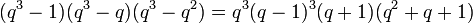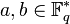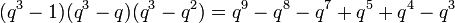# Element structure of general linear group of degree three over a finite field

(Redirected from GL(3,q) conjugacy classes)

## Contents

This article gives specific information, namely, element structure, about a family of groups, namely: general linear group of degree three.
View element structure of group families | View other specific information about general linear group of degree three

This article discusses the element structure of the general linear group of degree three over a finite field. The group is$GL(3,q)$ where$q$ is the order (size) of the field. We denote by$p$ the prime number that is the characteristic of the field.

## Particular cases

Group$p$$q$ Order of the group Number of conjugacy classes Element structure page
projective special linear group:PSL(3,2) 2 2 168 6 element structure of projective special linear group:PSL(3,2)
general linear group:GL(3,3) 3 3 11232 24 element structure of general linear group:GL(3,3)
general linear group:GL(3,5) 5 5 1488000 120 element structure of general linear group:GL(3,5)

## Conjugacy class structure

There is a total of$(q^3 - 1)(q^3 - q)(q^3 - q^2) = q^3(q - 1)^3(q + 1)(q^2 + q + 1)$ elements, and a total of$q^3 - q = q(q - 1)(q + 1)$ conjugacy classes.

Nature of conjugacy class Eigenvalues Characteristic polynomial Minimal polynomial Size of conjugacy class Number of such conjugacy classes Total number of elements Semisimple? Diagonalizable over$\mathbb{F}_q$?
Diagonalizable over$\mathbb{F}_q$ with equal diagonal entries, hence a scalar$\{a,a,a \}$ where$a \in \mathbb{F}_q^\ast$$(x - a)^3$$x - a$ 1$q - 1$$q - 1$ Yes Yes
Diagonalizable over$\mathbb{F}_q$ with one eigenvalue having multiplicity two, the other eigenvalue having multiplicity one$\{ a,a,b \}$ where$a \ne b$, both in$\mathbb{F}_q^\ast$$(x - a)^2(x - b)$$(x - a)(x - b)$$q^2(q^2 + q + 1) = q^4 + q^3 + q^2$$(q - 1)(q - 2)$$q^2(q^2 + q + 1)(q - 1)(q - 2) = q^6 - 2q^5 - q^3 + 2q^2$ Yes Yes
Diagonalizable over$\mathbb{F}_q$ with all distinct diagonal entries$\{ a,b,c \}$, all distinct elements of$\mathbb{F}_q^\ast$$(x - a)(x - b)(x - c)$ same as characteristic polynomial$q^3(q + 1)(q^2 + q + 1)$$(q-1)(q-2)(q-3)/6 = (q^3 - 6q^2 + 11q - 6)/6$$q^3(q + 1)(q^2 + q + 1)(q - 1)(q - 2)(q - 3)/6 = (q^9 - 4q^8 + q^7 + 5q^6 + 4q^5 - q^4 - 6q^3)/6$ Yes Yes
Diagonalizable over$\mathbb{F}_{q^3}$, not over$\mathbb{F}_q$ Distinct Galois conjugate triple of elements in$\mathbb{F}_{q^3}^\ast$. If one of the elements is$a$, the other two are$a^q$ and$a^{q^2}$. irreducible degree three polynomial over$\mathbb{F}_q$ same as characteristic polynomial$q^3(q - 1)^2(q + 1)$$q(q + 1)(q - 1)/3 = (q^3 - q)/3$$q^4(q - 1)^3(q + 1)^2/3 = (q^9 - q^8 - 2q^7 + 2q^6 + q^5 - q^4)/3$ Yes No
One eigenvalue is in$\mathbb{F}_q^\ast$, the other two are in$\mathbb{F}_{q^2} \setminus \mathbb{F}_q$ one element of$\mathbb{F}_q^\ast$, pair of Galois conjugates over$\mathbb{F}_q$ in$\mathbb{F}_{q^2}$. product of linear polynomial and irreducible degree two polynomial over$\mathbb{F}_q$ same as characteristic polynomial$q^3(q - 1)(q^2 + q + 1) = q^6 - q^3$$q(q - 1)^2/2 = (q^3 - 2q^2 + q)/2$$q^4(q - 1)^3(q^2 + q + 1)/2 = (q^9 - 2q^8 + q^7 - q^6 + 2q^5 - q^4)/2$ Yes No
Has Jordan blocks of sizes 2 and 1 with distinct eigenvalues over$\mathbb{F}_q$$\{ a,a,b \}$ with$a,b \in \mathbb{F}_q^\ast$,$a \ne b$$(x - a)^2(x - b)$ same as characteristic polynomial$q^2(q + 1)(q - 1)(q^2 + q + 1)$$(q - 1)(q - 2)$$q^2(q -1)^2(q + 1)(q^2 + q + 1)(q - 2) = q^8 - 2q^7 - q^6 + q^5 + 2q^4 + q^3 - 2q^2$ No No
Has Jordan blocks of sizes 2 and 1 with equal eigenvalues over$\mathbb{F}_q$$\{ a,a,a \}$ with$a \in \mathbb{F}_q^\ast$$(x - a)^3$$(x - a)^2$$q(q + 1)(q - 1)^2(q^2 + q + 1) = q^6 - q^4 - q^2 + q$$q - 1$$q(q + 1)(q - 1)^3(q^2 + q + 1) = q^7 - q^6 - q^5 + q^3 + q^2 - q$ No No
Has Jordan block of size 3$\{ a,a,a \}$ with$a \in \mathbb{F}_q^\ast$$(x - a)^3$ same as characteristic polynomial$(q - 1)(q + 1)(q^2 + q + 1) = q^4 + q^3 - q - 1$$q - 1$$(q - 1)^2(q + 1)(q^2 + q + 1) = q^5 - q^3 - q^2 + 1$ No No
Total NA NA NA NA$q^3 - q$$(q^3 - 1)(q^3 - q)(q^3 - q^2) = q^9 - q^8 - q^7 + q^5 + q^4 - q^3$ NA NA